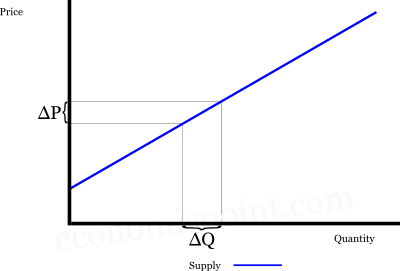# Supply Elasticity

When the price of a product or service increases (for example: if the demand increases), the quantity produced usually increases. Similarly, when the demand decreases, the price decreases and the quantity produced usually decreases.

The variation in the quantity in the face of a price variation can be big or small. But how to measure if the responsiveness of the supply is big or small?

The price elasticity of the supply measures the responsiveness of the quantity supplied, when the price variates. It is defined by the proportional change in the quantity supplied, divided the proportional change in the price:

es = (ΔQ/Q)/(ΔP/P)

Where:

• ΔQ = Variation in the quantity supplied
• ΔP = Variation in the price
• Q = quantity supplied
• P = price

Graphically:## How to Calculate Price Elasticity of Supply

### Percentaje Method

The percentaje method takes the initial pair of price and quantity as the base point for the calculation:

es = (ΔQ/Q)/(ΔP/P)

ΔQ = Q1 - Q
ΔP = P1 - P

Where:

• Q1 = Quantity after the price change
• Q0 = Quantity before the price change
• P0 = Old price
• P1 = New price

#### Numerical Example

The price of a product increases from 10 to 12. The quantity produced increases from 100 to 110. Using the percentaje method, the price elasticity of the supply is:

es = (10/100)/(2/10) = 0.1 / 0.2 = 0.5

### Arc Elasticity Method

The arc elasticity method takes the midpoint as base for the calculation:

es = (ΔQ/average quantity) (ΔP/average price)

es = (ΔQ/((Q+Q1)/2)/(ΔP/(P+P1)/2)

#### Numerical Example

The price of a product increases from 10 to 12. The quantity produced increases from 100 to 110. Using the percentaje method, the price elasticity of the supply is:

es = (10/105)/(2/11) = 0.524

The main difference between the percentaje method and the arc method is that, using the first one, the value of the supply elasticity will change depending if the price is increasing or decreasing. Using the arc method, the value of the elasticity will be the same independently if the price increases or decreases.

### Point Method

This method is used when we have a continuous supply function. A supply function is a mathematical relation between the quantity supplied and the price. Qs = f(P).

The price elasticity of the supply is:

es = (ΔQ/Q )/(ΔP/P )

And can be rewriten as:

es = (ΔQ/ΔP)/(Q /P )

If the supply is a continuous function, this can be rewriten as:

es = (dQ/dP)/(Q /P )

## Cross Elasticity of Supply

The cross elasticity of supply measures a proportional change in the quantity supplied in relation to the proportional change in the price.

e12 = ΔQs1/Qs1 / ΔP2/P2

Where:

• e12 : cross elasticity between goods 1 and 2
• ΔQs1 : change in quantity supplied of good 1
• ΔP2 : change in quantity supplied of good 2

Looking at the cross elasticity, we can see if 2 goods are substitutes or complements in production.

### Complements in Production

2 goods are complements in production when they are simultaneously produced using the same resources. For example: low fat milk and cream.

The cross elasticity of complements in production goods is positive: if the price of a complement in production increases, the supply of the other good increases. The producer will assign more resourcers for the production of both goods.

### Substitutes in Production

2 goods are substitutes in production when they use the same inputs, but the inputs can be assigned to the production of a good or another, not both simultaneously. For example: corn and soybeans.

The cross elasticity of substitutes in production goods is negative: if the price of a substitute in production increases, the supply of the other good decreases. The supplier will move resources from the production of a good to the other one.

## Determinants of Elasticity of Supply

• Long run vs short rung: the supply is more elastic in the long run.
• Avalilability of inputs: when the inputs are easily available, the supply is more elastic.
• Complexity of technolgy: if the technology is simple, the supply will be more elastic.